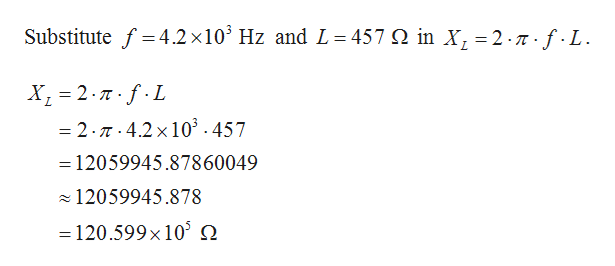solve the given formula for inductance. then calculate the inductance for an AC circuit with frequency of 4.2 KHz and an inductive reactance of 457 ohm. round to 3 decimal places.x= 2Pi fl

Question

solve the given formula for inductance. then calculate the inductance for an AC circuit with frequency of 4.2 KHz and an inductive reactance of 457 ohm. round to 3 decimal places.

x= 2Pi fl

Step 1

Given that, the frequency is f = 4.2 kHz and the inductance reactance is  L = 457 ohm.

It is known t...help_outlineImage TranscriptioncloseSubstitutef4.2 x 103 Hz and L 457 Q in X, = 2-n-f-L X1 2.TfL =2.7.4.2x 103 . 457 12059945.87860049 12059945.878 120.599x 105 . fullscreen

Want to see the full answer?

See Solution

Want to see this answer and more?

Our solutions are written by experts, many with advanced degrees, and available 24/7

See Solution
Tagged in

Other